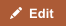Real GDP offers a better perspective than nominal GDP when tracking economic output over a period of time. When people use GDP numbers, they are often talking about nominal GDP, which can be defined as the total economic output of a country. This output is measured at current price levels and currency values, without factoring in inflation.

## Comparison chart

Nominal GDP versus Real GDP comparison chartNominal GDPReal GDP
Definition Nominal GDP is the market value (money-value) of all final goods and services produced in a geographical region, usually a country. Real GDP is a macroeconomic measure of the value of output economy, adjusted for price changes. The adjustment transforms the nominal GDP into an index for quantity of total output.
Also known as GDP Constant GDP

## Calculating real vs nominal GDP

Nominal GDP = ∑ ptqt

where p refers to price, q is quantity, and t indicates the year in question (usually the current year).

However, it can be misleading to do an apples-to-apples comparison of a GDP of \$1 trillion in 2008 with a GDP of \$200 billion in 1990. This is because of inflation. The value of one dollar in 1990 was far greater than the value of a dollar in 2008. In other words, prices in 1990 were different from prices in 2008. So if you want to really compare economic output (quantities), you can calculate GDP by using prices from a base year.

When you adjust nominal GDP for price changes (inflation or deflation), you get what is known as the Real GDP. It can be calculated using the following formula:

Real GDP = ∑ pbqt

where b denotes the base year.

To effectively compare the real GDP of two years, one can construct an index using a base year. A base year is usually an arbitrary figure (here, a particular year) which is used as a yardstick for comparison of the GDP numbers.

## Usage and Applications

When should we use real GDP numbers and when is nominal GDP used?

One uses the nominal GDP figures to determine the total value of the products and services manufactured in a country during a particular year. However, when one wants to compare GDP in one year with past years to study trends in economic growth, real GDP is used.

By definition (since real GDP is calculated using prices of a given "base year"), real GDP has no meaning by itself unless it is compared to GDP of a different year.

If a set of real GDPs from various years are calculated, each calculation uses the quantities from its own year, but all use the prices from the same base year. The differences in those real GDPs will, therefore, reflect merely differences in volume.

An index called the GDP deflator can be obtained by dividing, for each year, the nominal GDP by the real GDP. It gives an indication of the overall level of inflation or deflation in the economy.

GDP deflator for year t = GDPt / Real GDPt

## Top Countries by GDP

In terms of nominal GDP, the top five countries are:

1. United States of America
2. China
3. Japan
4. Germany
5. France

### Countries by growth rates in real GDP

If there is high inflation in a country, there may be rapid growth in nominal GDP but not much growth in real GDP. All countries have different rates of inflation. Therefore, when comparing GDP growth rates in different countries, real GDP is used and not nominal GDP. Real GDP growth paints a more accurate picture and allows economists to compare economic growth in different countries. The top five countries in terms of real GDP growth rate for 2009 were:

1. Macau
2. Qatar
3. Azerbaijan
4. China
5. Ethiopia

## References

Share this comparison:

If you read this far, you should follow us:

"Nominal GDP vs Real GDP." Diffen.com. Diffen LLC, n.d. Web. 20 Jun 2021. < >